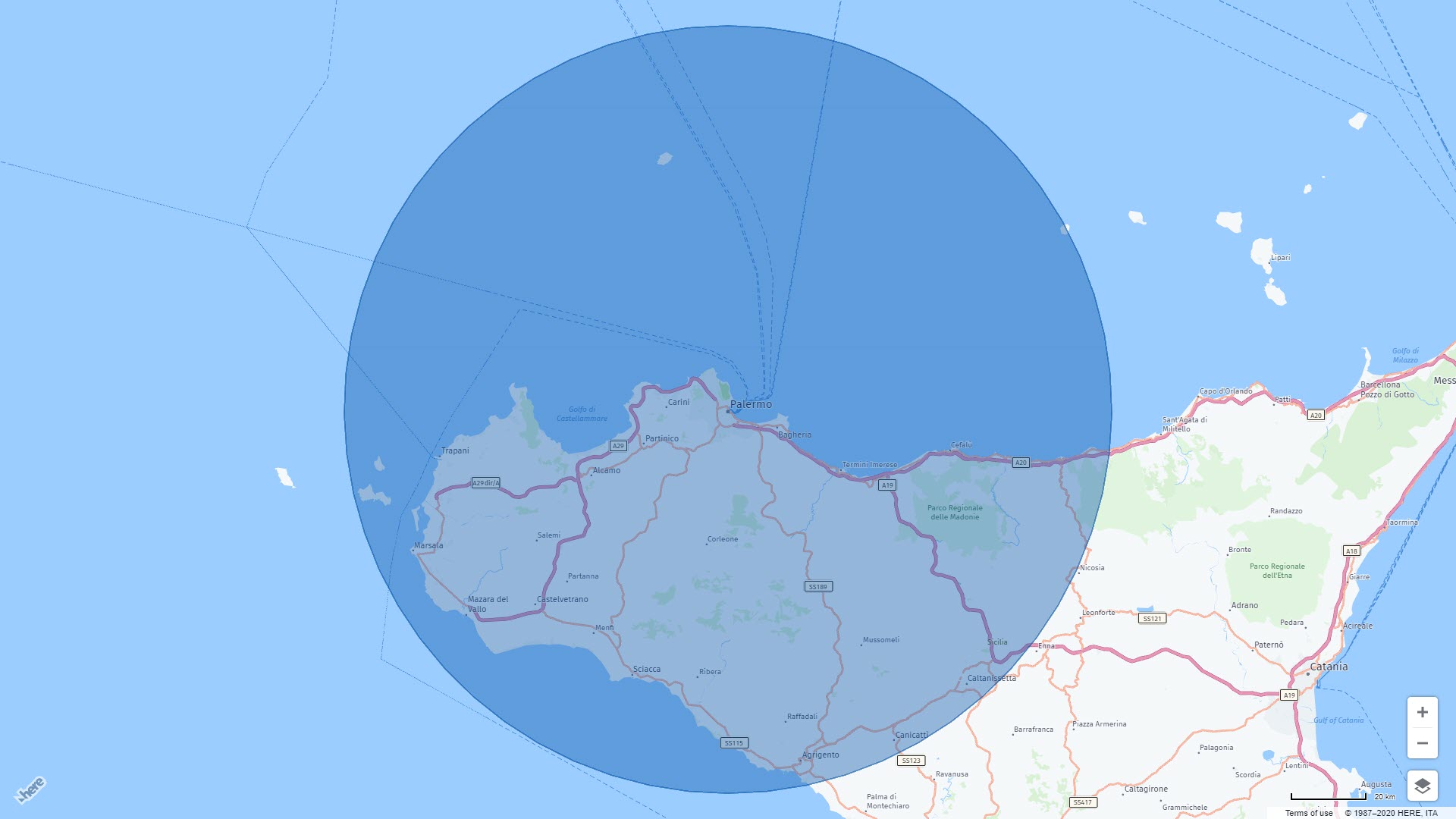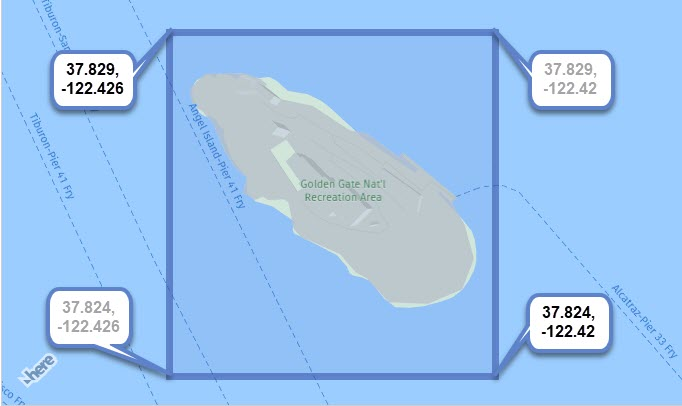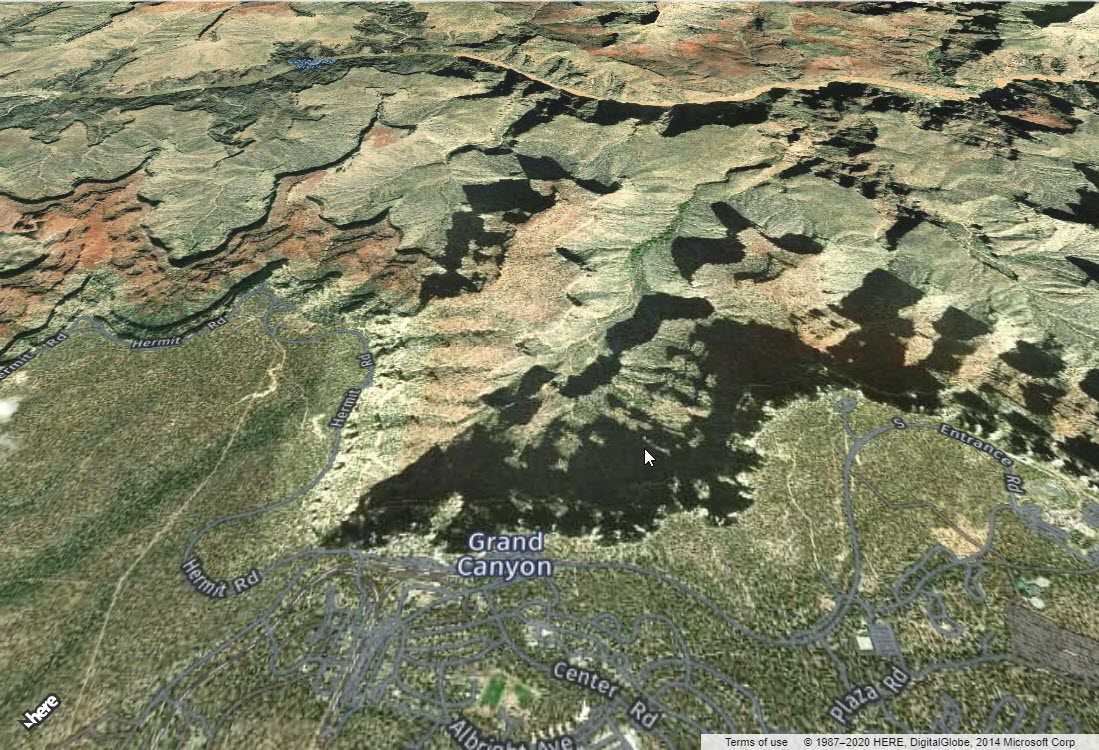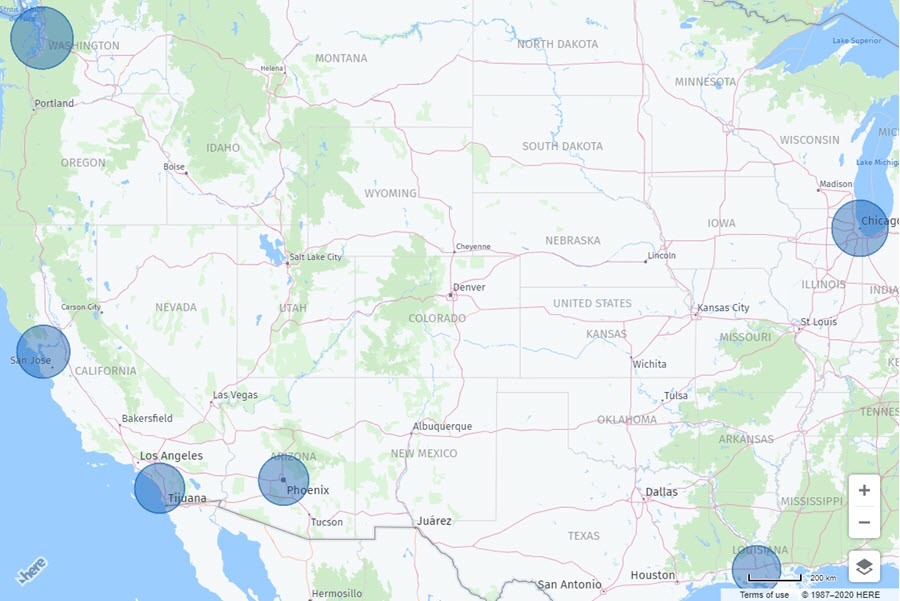Hands On

# Get the Most Out of Bounds

By Michael Palermo | 11 June 2020### Try HERE Maps

Create a free API key to build location-aware apps and services.

The world is round, yet most maps are flat rectangles. When we are interested in a certain area of a map, we may narrow our focus to that region of the map. With digital maps, we can even zoom in for details. As a developer with HERE, you can create compelling user experiences surrounding these areas or regions, by using bounding boxes. In this post, you will learn the concept of a bounding box, and how to implement with a few scenarios.

### What is a Bounding Box?

To understand a "bounding box" we will first consider what the "box" is. To understand the concept, consider the following map image:A blue rectangle is shown to help demonstrate the concept. Each corner has coordinates. The upper left corner coordinates and lower right corner coordinates (shown with bolder font) are all that is needed to create this area on the map. In the example above, the "box" is visible because a rectangle was drawn there, but in many uses of a bounding box, you will never see a border. You will soon see why.

And what about the "bounding" part of the concept? In the example above, the box is bound to static coordinates provided by the developer. We will see other types of bindings later in this post.

### Controlling the Map with Bounds

In the following code example, a map of a specific region of the Grand Canyon is bound to the viewing area of the map.


// get reference to map container
let container = document.getElementById('map');

// initialized platform
let platform = new H.service.Platform(
{apikey: "your-apikey"}
);

// get reference to layers/map types
let layers = platform.createDefaultLayers();

// initialize map with container and map type
let map = new H.Map(container, layers.raster.satellite.map, {
// No need to add coordinates or zoom level
pixelRatio: window.devicePixelRatio || 1
});

// To make the map static, do not add these lines...
// let behavior = new H.mapevents.Behavior(new H.mapevents.MapEvents(map));
// let ui = H.ui.UI.createDefault(map, layers);

// when screen resizes, resize map container

// initialize
(()=>{
let GC_Bounds = new H.geo.Rect(36.08536, -112.14056, 36.04881, -112.13);
// change map bounds to rectangle coordinates at 45 degree tilt
map.getViewModel().setLookAtData({
bounds: GC_Bounds,
tilt: 45
});
})();


The above code turns off all interactions and UI controls, so only the exact area specified in the coordinates area dominate on the screen as shown here:This is a simple starting place. We used the bounding box of a rectangle (no visible border) created with static coordinates to determine what would be shown in the map. Let's look at other uses.

### Bounding to an Object

In the following initializing code, a circle object is added to the map. When the circle is tapped/clicked, the map view is changed to the bounding box of the circle.


// initialize
(()=>{
// create a circle with centered at Palermo, Sicily
let circle = new H.map.Circle( {lat:38.12207,lng:13.36112}, 98765);
// add event handler for when the circle is tapped or clicked
// get instance of circle via evt.target
let target = evt.target;
// set the map dimensions to bounding box of circle
map.getViewModel().setLookAtData({
bounds: target.getBoundingBox()
});
});
})();


So when the map is first displayed, the circle may appear small over Palermo, Sicily. By clicking the circle, the map view is then bound to the invisible bounding box surrounding the circle so that it will occupy most of the screen as shown here:As a developer, you did not need to calculate the region or box surrounding the circle, just calling the getBoundingBox() method did all the work for you. Lets now look at another example.

### Bounding to a Group

In the next scenario, many circles are added to the map. This time, the circles are added to a group, then the group is added to the map. This makes it possible to get the bounding box of the group, and bind it to the view area of the map. In other words, fit the map to show all the circles. Consider the following code:


// initialize
(()=>{
// create a group
let group = new H.map.Group();

// ...
// after all circle objects are added to group...
// ...

// change map to focus in an all circles in group
map.getViewModel().setLookAtData({
bounds: group.getBoundingBox()
});
})();


The result of the above code is a map starting with a view containing all the circles as shown here:Each circle on the map above belongs to a group, and the bounding box of that group is what the map is now revealing.

### Summary

In this post, we learned the concept of a bounding box, and how it is bound to coordinates, a single object, or a group of objects. Want to see these examples demonstrated? Check out our video below!